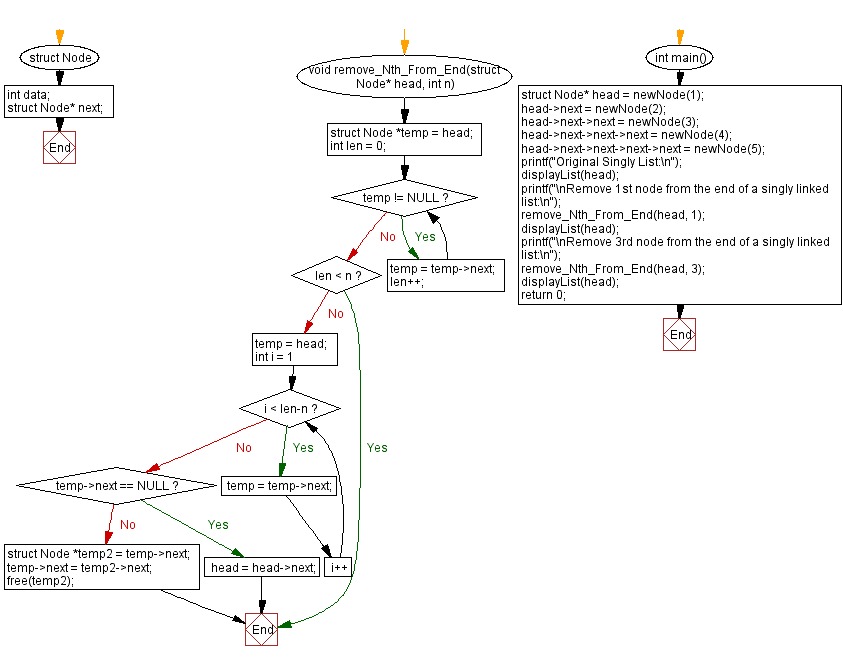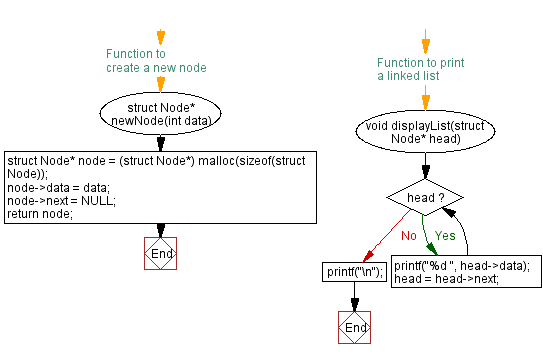﻿ C Program: Remove Nth node from the end of a singly linked list

# C Exercises: Remove Nth node from the end of a singly linked list

## C Singly Linked List : Exercise-28 with Solution

Write a C program to remove the Nth node from the end of a singly linked list.

Sample Solution:

C Code:

``````#include<stdio.h>
#include <stdlib.h>

struct Node {
int data;
struct Node* next;
};

// Function to create a new node
struct Node* newNode(int data) {
struct Node* node = (struct Node*) malloc(sizeof(struct Node));
node->data = data;
node->next = NULL;
return node;
}

void remove_Nth_From_End(struct Node* head, int n){
int len = 0;
while(temp != NULL){
temp = temp->next;
len++;
}
if(len < n){
return;
}
for(int i = 1; i < len-n; i++){
temp = temp->next;
}
if(temp->next == NULL){
return;
}
struct Node *temp2 = temp->next;
temp->next = temp2->next;
free(temp2);
}
// Function to print a linked list
}
printf("\n");
}

int main() {
printf("Original Singly List:\n");
printf("\nRemove 1st node from the end of a singly linked list:\n");
printf("\nRemove 3rd node from the end of a singly linked list:\n");
return 0;
}
```
```

Sample Output:

```Original Singly List:
1 2 3 4 5

Remove 1st node from the end of a singly linked list:
1 2 3 4

Remove 3rd node from the end of a singly linked list:
1 3 4
```

Flowchart :C Programming Code Editor:

What is the difficulty level of this exercise?

﻿